Documentation

## Modeling Probabilities of Default with Cox Proportional Hazards

This example shows how to work with consumer (retail) credit panel data to visualize observed probabilities of default (PDs) at different levels. It also shows how to fit a Cox proportional hazards (PH) model, also known as Cox regression, to predict PDs. In addition, it shows how to perform a stress-testing analysis and how to model lifetime PDs and the lifetime Expected Credit Loss (ECL) value.

A similar example, Stress Testing of Consumer Credit Default Probabilities Using Panel Data, follows the same workflow but uses logistic regression instead of Cox PH. The main differences in the two approaches are:

• The model fit: The Cox PH model has a nonparametric baseline hazard rate that can match patterns in the PDs much more closely than the fully parametric logistic model.

• Extrapolating beyond the observed ages in the data: The Cox PH model, because it is built on top of a nonparametric baseline hazard rate, cannot extrapolate to loan ages that are not observed in the data set. The logistic model treats the age of the loan as a continuous variable, and, therefore, it can extrapolate to predict PDs for ages not observed in the data set.

• Low probability situations: If, for a particular age, the PD is small and there are no observed defaults in the data, the Cox PH model predicts the PD as zero. In contrast, the logistic model always gives nonzero probabilities.

### Data Exploration with Survival Analysis Tools

Starting with some data visualizations, mainly the visualization of PDs as a function of age, which in this data set is the same as Years-on-Books (YOB). Because Cox PH is a survival analysis model, this example discusses some survival analysis tools and concepts and uses the empirical cumulative distribution function (`ecdf`) functionality for some of these computations and visualizations.

The main data set (`data`) contains the following variables:

• `ID`: Loan identifier.

• `ScoreGroup`: Credit score at the beginning of the loan, discretized into three groups: `High Risk`, `Medium Risk`, and `Low Risk`.

• `YOB`: Years on books.

• `Default`: Default indicator. This is the response variable.

• `Year`: Calendar year.

There is also a small data set (`dataMacro`) with macroeconomic data for the corresponding calendar years:

• `Year`: Calendar year.

• `GDP`: Gross domestic product growth (year over year).

• `Market`: Market return (year over year).

The variables `YOB`, `Year`, `GDP`, and `Market` are observed at the end of the corresponding calendar year. The score group is a discretization of the original credit score when the loan started. A value of `1` for `Default` means that the loan defaulted in the corresponding calendar year.

There is also a third data set (`dataMacroStress`) with baseline, adverse, and severely adverse scenarios for the macroeconomic variables. This table is used for the stress-testing analysis.

Load the simulated data.

```load RetailCreditPanelData.mat disp(head(data,10))```
``` ID ScoreGroup YOB Default Year __ ___________ ___ _______ ____ 1 Low Risk 1 0 1997 1 Low Risk 2 0 1998 1 Low Risk 3 0 1999 1 Low Risk 4 0 2000 1 Low Risk 5 0 2001 1 Low Risk 6 0 2002 1 Low Risk 7 0 2003 1 Low Risk 8 0 2004 2 Medium Risk 1 0 1997 2 Medium Risk 2 0 1998 ```

Preprocess the panel data to put it in the format expected by some of the survival analysis tools.

```% Use groupsummary to reduce data to one ID per row, and keep track of whether the loan defaulted or not dataSurvival = groupsummary(data,'ID','sum','Default'); disp(head(dataSurvival,10))```
``` ID GroupCount sum_Default __ __________ ___________ 1 8 0 2 8 0 3 8 0 4 6 0 5 7 0 6 7 0 7 8 0 8 6 0 9 7 0 10 8 0 ```
```% Could also get years observed from YOB, though here we know YOB always % starts from 1 in the data, so the GroupCount equals the final YOB dataSurvival.Properties.VariableNames{2} = 'YearsObserved'; dataSurvival.Properties.VariableNames{3} = 'Default'; % If there is no default, it's a censored observation dataSurvival.Censored = ~dataSurvival.Default; disp(head(dataSurvival,10))```
``` ID YearsObserved Default Censored __ _____________ _______ ________ 1 8 0 true 2 8 0 true 3 8 0 true 4 6 0 true 5 7 0 true 6 7 0 true 7 8 0 true 8 6 0 true 9 7 0 true 10 8 0 true ```

The main variable is the amount of time each loan was observed (`YearsObserved`), which is the final value of the Years-on-Books (`YOB`) variable. This is the number of years until default, or until the end of the observation period (eight years), or until the loan is removed from the sample, for example, due to prepayment. In this data set, the YOB information is the same as the age of the loan, because all loans start with a YOB of `1`. For other data sets this might not be the case. In a trading portfolio, YOB and age may be different, because a loan purchased in the third year of its life would have an age of `3`, but a YOB value of `1`.

The second required variable is the censoring variable (`Censored`). The event of interest is default in this analysis. If a loan is observed until default, we have full information about the time until default, therefore the lifetime information is uncensored or complete. Alternatively, the information is considered censored, or incomplete, if at the end of the observation period the loan has not defaulted. This could be because the loan was prepaid, or because the loan had not defaulted by the end of the eight-year observation period in the sample.

Add the score group and vintage information to the data. The value of these variables remains constant throughout the life of the loan. The score given at origination determines the score group and the origination year determines the vintage or cohort.

```% Can get ScoreGroup from YOB==1, since we know in this data set % YOB always starts at 1 and the ID's order is the same in data and dataSurvival dataSurvival.ScoreGroup = data.ScoreGroup(data.YOB==1); % Define vintages based on the year the loan started, we know all loans % in this data set start in year 1 of their life dataSurvival.Vintage = data.Year(data.YOB==1); disp(head(dataSurvival,10))```
``` ID YearsObserved Default Censored ScoreGroup Vintage __ _____________ _______ ________ ___________ _______ 1 8 0 true Low Risk 1997 2 8 0 true Medium Risk 1997 3 8 0 true Medium Risk 1997 4 6 0 true Medium Risk 1999 5 7 0 true Medium Risk 1998 6 7 0 true Medium Risk 1998 7 8 0 true Medium Risk 1997 8 6 0 true Medium Risk 1999 9 7 0 true Low Risk 1998 10 8 0 true Low Risk 1997 ```

Finally, compare the number of rows in the original data set, in panel data format, and the aggregated data set, in the more traditional survival format.

`fprintf('Number of rows original data: %d\n',height(data));`
```Number of rows original data: 646724 ```
`fprintf('Number of rows survival data: %d\n',height(dataSurvival));`
```Number of rows survival data: 96820 ```

Plot the cumulative default probability against YOB for the entire portfolio (all score groups and vintages) using the empirical cumulative distribution function (`ecdf`) functionality.

```ecdf(dataSurvival.YearsObserved,'Censoring',dataSurvival.Censored,'Bounds','on') title('Cumulative Default Probability, All Score Groups') xlabel('Years on Books')```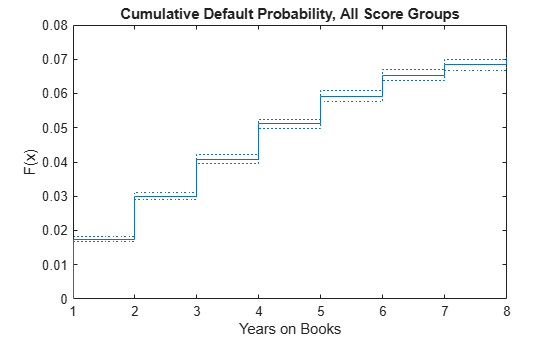Plot conditional one-year PDs against YOB. For example, the conditional one-year PD for a YOB of 3 is the conditional one-year PD for loans that are in their third year of life. In survival analysis, this is called the discrete hazard rate, denoted by h. To compute h, get the cumulative hazard function output, denoted by H, and transform it to the hazard function h.

```[H,x] = ecdf(dataSurvival.YearsObserved,'Censoring',dataSurvival.Censored,... 'Function','cumulative hazard'); % Because it is discrete time, simply take the diff of H to get the hazard h h = diff(H); x(1) = []; % In this example, the times observed (stored in variable x) do not change for % different score groups, or for training vs. test sets. For other data sets, % the x and h variables may need to be checked after every call to the ecdf function before % plotting or concatenating results (for example, if there are no defaults in a % particular year for the test data). plot(x,h,'*') grid on title('Conditional 1-Year PDs') ylabel('PD') xlabel('Years on Books')```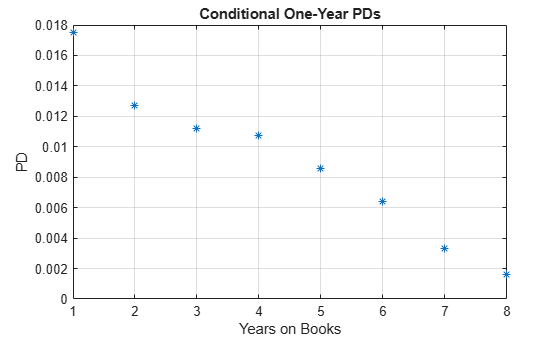You can also compute these probabilities directly with `groupsummary` using the original panel data format. For more information, see the companion example Stress Testing of Consumer Credit Default Probabilities Using Panel Data. Or you can compute these probabilities with `grpstats` using the original panel data format. These approaches give the same conditional one-year PDs.

```PDvsYOBByGroupsummary = groupsummary(data,'YOB','mean','Default'); PDvsYOBByGrpstats = grpstats(data.Default,data.YOB); PDvsYOB = table((1:8)',h,PDvsYOBByGroupsummary.mean_Default,PDvsYOBByGrpstats,... 'VariableNames',{'YOB','ECDF','Groupsummary','Grpstats'}); disp(PDvsYOB)```
``` YOB ECDF Groupsummary Grpstats ___ _________ ____________ _________ 1 0.017507 0.017507 0.017507 2 0.012704 0.012704 0.012704 3 0.011168 0.011168 0.011168 4 0.010728 0.010728 0.010728 5 0.0085949 0.0085949 0.0085949 6 0.006413 0.006413 0.006413 7 0.0033231 0.0033231 0.0033231 8 0.0016272 0.0016272 0.0016272 ```

Segment the data by score group to get the PDs disaggregated by score group.

```ScoreGroupLabels = categories(dataSurvival.ScoreGroup); NumScoreGroups = length(ScoreGroupLabels); hSG = zeros(length(h),NumScoreGroups); for ii=1:NumScoreGroups Ind = dataSurvival.ScoreGroup==ScoreGroupLabels{ii}; H = ecdf(dataSurvival.YearsObserved(Ind),'Censoring',dataSurvival.Censored(Ind)); hSG(:,ii) = diff(H); end plot(x,hSG,'*') grid on title('Conditional 1-Year PDs, By Score Group') xlabel('Years on Books') ylabel('PD') legend(ScoreGroupLabels)```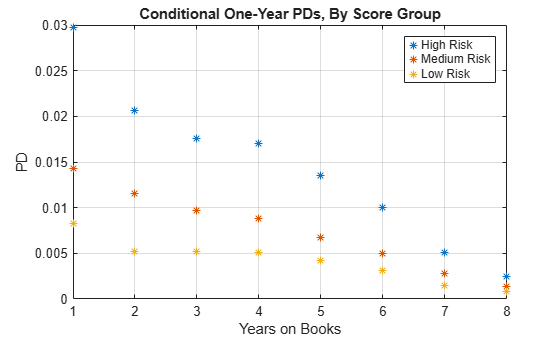You can disaggregate PDs by vintage and segment the data accordingly in a similar way. You can plot these PDs against YOB or against calendar year. To see these visualizations, refer to Stress Testing of Consumer Credit Default Probabilities Using Panel Data.

### Cox PH Model Without Macro Effects

This section shows how to fit a Cox PH model without macro information. For this, the survival data format is used and the model includes only time-independent predictors, that is, information that remains constant throughout the life of the loan. Only the score group at the origination of the loans is used as a time-independent predictor, but other such predictors could be added to the model (for example, vintage information).

Cox proportional hazards regression is a semiparametric method for adjusting survival rate estimates to quantify the effect of predictor variables. The method represents the effects of explanatory variables as a multiplier of a common baseline hazard function, ${\mathit{h}}_{0}\left(\mathit{t}\right)$. The hazard function is the nonparametric part of the Cox proportional hazards regression function, whereas the impact of the predictor variables is a loglinear regression. This Cox PH model is

`$\mathit{h}\left({\mathit{X}}_{\mathit{i}},\mathit{t}\right)={\mathit{h}}_{0}\left(\mathit{t}\right)\mathrm{exp}\left(\sum _{\mathit{j}=1}^{\mathit{p}}{\mathit{x}}_{\mathrm{ij}}{\mathit{b}}_{\mathit{j}}\right)$`

where:

• ${\mathit{X}}_{\mathit{i}}=\left({\mathit{x}}_{\mathrm{i1}},...,{\mathit{x}}_{\mathrm{ip}}\right)$ are the predictor variables for the ith subject.

• ${\mathit{b}}_{\mathit{j}}$ is the coefficient of the jth predictor variable.

• $\mathit{h}\left({\mathit{X}}_{\mathit{i}},\mathit{t}\right)$ is the hazard rate at time t for ${\mathit{X}}_{\mathit{i}}$.

• ${\mathit{h}}_{0}\left(\mathit{t}\right)$ is the baseline hazard rate function.

For more details, see `coxphfit` or the Cox Proportional Hazards Model and the references therein.

The basic Cox PH model assumes that the predictor values do not change throughout the life of the loans. In our example, this is the case for the score group, because it is the score given to borrowers at the beginning of the loan. The vintage is also constant throughout the life of the loan.

A model could use time-dependent scores, for example, if the credit score information were updated every year. In this case, you model a time-dependent predictor in the Cox PH model, similar to the way the macro variables are added to the model later in the "Cox PH Model with Macro Effects" section.

To assess the model fit, visualize the in-sample and out-of-sample fit of the model. First, split the data into training and testing subsets, and fit the model using the training data.

```nIDs = height(dataSurvival); NumTraining = floor(0.6*nIDs); % Use 60% of data for training rng('default'); % Reset rng state, for reproducibility TrainIDInd = randsample(nIDs,NumTraining); TrainDataInd = ismember(dataSurvival.ID,TrainIDInd); TestDataInd = ~TrainDataInd; % ScoreGroup is categorical, convert to binary columns with dummyvar X = dummyvar(dataSurvival.ScoreGroup(TrainDataInd)); % Drop first column to avoid linear dependencies, % also known as the "dummy variable trap" X(:,1) = []; % Fit the Cox PH model [bCox,~,HCox] = coxphfit(X,... dataSurvival.YearsObserved(TrainDataInd),... 'Censoring',dataSurvival.Censored(TrainDataInd),... 'Baseline',0);```

The third output of the `coxphfit` function is the baseline cumulative hazard rate H. This cumulative hazard rate can be converted to the hazard rate h as before, except for an additional step. The Cox PH model assumes that the observation time is measured as a continuous variable. Here, the time is measured in a discrete way, because the time is always a whole number between `1` and `8`. The `coxphfit` function supports ways to handle ties in the time variable (see the `'Ties'` optional input in `coxphfit`). Because of the ties, the H output has multiple rows with the same YOB values, but it can be processed to have only as many rows as the unique YOB values, as shown here.

```% Process baseline HCox to account for discrete time (remove time ties) HCoxDiscreteT = unique(HCox(:,1)); HCoxDiscreteH = grpstats(HCox(:,2),HCox(:,1),'max'); HCox = [HCoxDiscreteT HCoxDiscreteH]; % Convert baseline to h xCox = HCox(:,1); hCox = diff([0;HCox(:,2)]);```

Predict PDs, that is, hazard rates based on the model fit. Because this model uses only the initial score group information that is kept constant throughout the life of the loan. All loans with the same initial score group have the same predicted lifetime PDs. To get the aggregated predicted PD for the portfolio, compute the hazard rate for each score group. Then take the weighted average of these score groups, based on the proportion of loans in each score group in the training data.

The predicted PDs are compared against the observed PDs in the training data.

```% Compute proportion of loans in each score group ScoreGroupFraction = countcats(dataSurvival.ScoreGroup(TrainDataInd)); ScoreGroupFraction = ScoreGroupFraction/sum(ScoreGroupFraction); % Baseline h is the hazard rate for 'High Risk', in the first column % Columns 2 and 3 have the 'Medium' and 'Low' risk respectively, % which are adjusted by their corresponding parametric term of the Cox model hPredictedByScore = zeros(length(hCox),NumScoreGroups); hPredictedByScore(:,1) = hCox; for ii=2:NumScoreGroups hPredictedByScore(:,ii) = hCox*exp(bCox(ii-1)); end hPredicted = hPredictedByScore*ScoreGroupFraction; % Get the ecdf of the training data HTrain = ecdf(dataSurvival.YearsObserved(TrainDataInd),... 'Censoring',dataSurvival.Censored(TrainDataInd),... 'Function','cumulative hazard'); hTrain = diff(HTrain); plot(x,hPredicted,'-o',x,hTrain,'*') xlabel('Years on Books') ylabel('PD') legend('Model','Training') title('Model Fit (Training Data)') grid on```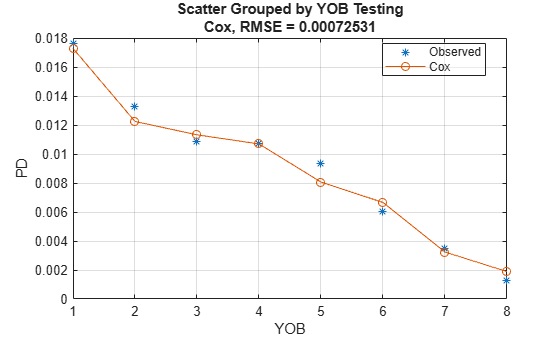Compare predicted vs. observed PDs in the test data.

```ScoreGroupFraction = countcats(dataSurvival.ScoreGroup(TestDataInd)); ScoreGroupFraction = ScoreGroupFraction/sum(ScoreGroupFraction); hPredicted = hPredictedByScore*ScoreGroupFraction; % Get the ecdf of the training data HTest = ecdf(dataSurvival.YearsObserved(TestDataInd),... 'Censoring',dataSurvival.Censored(TestDataInd),... 'Function','cumulative hazard'); hTest = diff(HTest); plot(x,hPredicted,'-o',x,hTest,'*') xlabel('Years on Books') ylabel('PD') legend('Model','Test') title('Model Fit (Test Data)') grid on```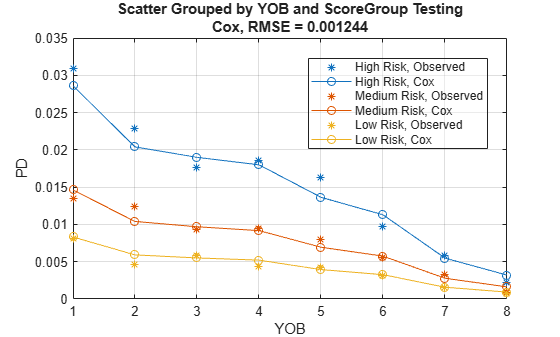The nonparametric part of the Cox PH model allows it to match the training data pattern closely, even using only score group information in this model. The out-of-sample errors (model vs. test data) are also small.

The addition of macro information is still important because both the stress testing and the lifetime PD projections require an explicit dependency on macro information.

### Cox PH Model with Macro Effects

This section shows how to fit a Cox PH model including macro information, specifically, gross domestic product (GDP) growth, and stock market growth. The value of the macro variables changes every year, therefore these are time-dependent predictors. The data input for the Cox PH functionality with time-dependent predictors uses the original panel data, with the addition of the macro information, and a time interval for each row.

The extension of the Cox proportional hazards model to account for time-dependent variables is

`$\mathit{h}\left({\mathit{X}}_{\mathit{i}},\mathit{t}\right)={\mathit{h}}_{0}\left(\mathit{t}\right)\mathrm{exp}\left(\sum _{\mathit{j}=1}^{\mathrm{p1}}{\mathit{x}}_{\mathrm{ij}}{\mathit{b}}_{\mathit{j}}+\sum _{\mathit{k}=1}^{\mathrm{p2}}{\mathit{x}}_{\mathrm{i}\mathit{k}}\left(\mathit{t}\right){\mathit{c}}_{\mathit{k}}\right)$`

where:

• ${\mathit{x}}_{\mathrm{ij}}$ is the predictor variable value for the ith subject and the jth time-independent predictor.

• ${\mathit{x}}_{\mathrm{i}\mathit{k}}\left(\mathit{t}\right)$ is the predictor variable value for the ith subject and the kth time-dependent predictor at time t.

• ${\mathit{b}}_{\mathit{j}}$ is the coefficient of the jth time-independent predictor variable.

• ${\mathit{c}}_{\mathit{k}}$ is the coefficient of the kth time-dependent predictor variable.

• $\mathit{h}\left({\mathit{X}}_{\mathit{i}}\left(\mathit{t}\right),\mathit{t}\right)$ is the hazard rate at time t for ${\mathit{X}}_{\mathit{i}}\left(\mathit{t}\right).$

• ${\mathit{h}}_{0}\left(\mathit{t}\right)$ is the baseline hazard rate function.

For more details, see `coxphfit` or the Cox Proportional Hazards Model and the references therein.

Macro variables are treated as time-dependent variables. If the time-independent information, such as the initial score group, provide a baseline level of risk through the life of the loan, it is reasonable to expect that changing macro conditions may increase or decrease the risk around that baseline level, and this variation would be different from one year to the next if the macro conditions change. For example, years with low economic growth should make all loans more risky, independently of their initial score group.

For the time-dependent Cox PH analysis, use an expansion of the original data set as input for the time-dependent analysis.

The tools expect the specification of time intervals, with the corresponding values of predictors. For the first year, the time interval is from 0 to 1, for the second year the time interval goes from 1 to 2, and so on. Therefore, the time interval range for each row is YOB-1 to YOB.

The time-independent variables have constant values throughout the history of the loans, as is the case for the score group information in the original data. No need to change anything for time-independent variables.

For time-dependent variables, the values change from one interval to the next. We add the GDP and Market variables as time-dependent predictors.

The tools also need a censoring flag, which is the negation of the default information.

```data.TimeInterval = [data.YOB-1 data.YOB]; data.GDP = dataMacro.GDP(data.Year-min(data.Year)+1); data.Market = dataMacro.Market(data.Year-min(data.Year)+1); data.Censored = ~data.Default; disp(head(data,10))```
``` ID ScoreGroup YOB Default Year TimeInterval GDP Market Censored __ ___________ ___ _______ ____ ____________ _____ ______ ________ 1 Low Risk 1 0 1997 0 1 2.72 7.61 true 1 Low Risk 2 0 1998 1 2 3.57 26.24 true 1 Low Risk 3 0 1999 2 3 2.86 18.1 true 1 Low Risk 4 0 2000 3 4 2.43 3.19 true 1 Low Risk 5 0 2001 4 5 1.26 -10.51 true 1 Low Risk 6 0 2002 5 6 -0.59 -22.95 true 1 Low Risk 7 0 2003 6 7 0.63 2.78 true 1 Low Risk 8 0 2004 7 8 1.85 9.48 true 2 Medium Risk 1 0 1997 0 1 2.72 7.61 true 2 Medium Risk 2 0 1998 1 2 3.57 26.24 true ```

Fit the time-dependent model. The same IDs as before belong to training or testing subsets, however, the indexing is different, because of the different formats in the data.

```TrainDataIndTD = ismember(data.ID,TrainIDInd); TestDataIndTD = ~TrainDataIndTD; XTD = dummyvar(data.ScoreGroup(TrainDataIndTD)); XTD(:,1) = []; [bCoxTD,~,HCoxTD] = ... coxphfit([XTD data.GDP(TrainDataIndTD) data.Market(TrainDataIndTD)],... data.TimeInterval(TrainDataIndTD,:),... 'Censoring',data.Censored(TrainDataIndTD),... 'Baseline',0); % Process baseline HCoxTD to account for discrete time (remove time ties) HCoxTDDiscreteT = unique(HCoxTD(:,1)); HCoxTDDiscreteH = grpstats(HCoxTD(:,2),HCoxTD(:,1),'max'); HCoxTD = [HCoxTDDiscreteT HCoxTDDiscreteH]; % Convert cumulative baseline to hCoxTD xCoxTD = HCoxTD(:,1); hCoxTD = diff([0;HCoxTD(:,2)]);```

To visualize the in-sample fit, compute the predicted PDs row by row. Set up the predictor matrix, as when fitting the model, and then apply the Cox PH formula is applied.

The macro effects help the model match the observed default rates even closer and the match to the training data almost looks like an interpolation for the macro model.

```% Set up predictor matrix XPredict = [dummyvar(data.ScoreGroup(TrainDataIndTD)), data.GDP(TrainDataIndTD), data.Market(TrainDataIndTD)]; XPredict(:,1) = []; % Predict at row level hPredictedTD = hCoxTD(data.YOB(TrainDataIndTD)).*exp(XPredict*bCoxTD); % Take the average of the predicted hazard rates at each time point (YOB) hPredictedTD = grpstats(hPredictedTD,data.YOB(TrainDataIndTD)); plot(x,hPredictedTD,'-o',x,hTrain,'*') xlabel('Years on Books') ylabel('PD') legend('Model','Training') title('Macro Model Fit (Training Data)') grid on```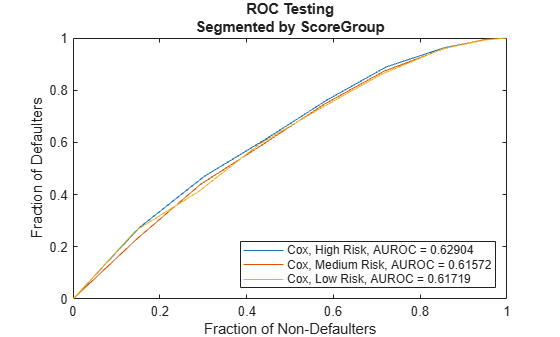Visualize the out-of-sample fit.

```% Set up predictor matrix XPredict = [dummyvar(data.ScoreGroup(TestDataIndTD)), data.GDP(TestDataIndTD), data.Market(TestDataIndTD)]; XPredict(:,1) = []; % Predict at row level hPredictedTD = hCoxTD(data.YOB(TestDataIndTD)).*exp(XPredict*bCoxTD); % Take the average of the predicted hazard rates at each time point (YOB) hPredictedTD = grpstats(hPredictedTD,data.YOB(TestDataIndTD)); plot(x,hPredictedTD,'-o',x,hTest,'*') xlabel('Years on Books') ylabel('PD') legend('Model','Test') title('Macro Model Fit (Test Data)') grid on```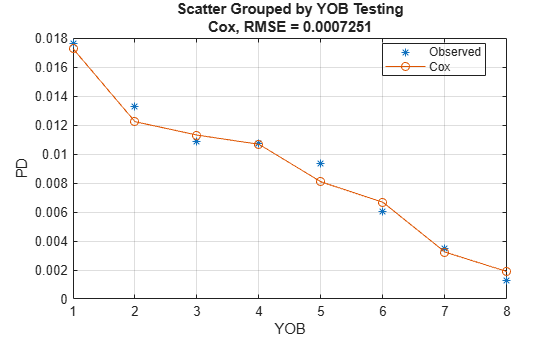To visualize the in-sample and out-of-sample at score group level, perform the same operations after segmenting the data by score group.

### Stress Testing

This section shows how to perform a stress testing analysis of PDs using the Cox PH macro model.

Assume that the following are stress scenarios for the macroeconomic variables provided, for example, by a regulator.

`disp(dataMacroStress)`
``` GDP Market _____ ______ Baseline 2.27 15.02 Adverse 1.31 4.56 Severe -0.22 -5.64 ```

The hazard rate, which gives the PD by YOB, is predicted for each group and each macro scenario. This code predicts PDs for each score group and each macro scenario.

For the visualization of each macro scenario, take the average over the score groups to aggregate into a single PD by YOB. The plot shows the predicted effect of adverse and severely adverse macro conditions on the average PD for each YOB.

```ScenarioLabels = dataMacroStress.Properties.RowNames; NumScenarios = length(ScenarioLabels); PDScenarios = zeros(length(x),NumScenarios); for ii=1:NumScoreGroups Score = ScoreGroupLabels{ii}; XPredictScore = ismember(ScoreGroupLabels(2:end)',Score); PDScenariosGroup = zeros(length(x),length(ScenarioLabels)); for jj=1:NumScenarios Scenario = ScenarioLabels{jj}; XPredictST = [XPredictScore dataMacroStress.GDP(Scenario) dataMacroStress.Market(Scenario)]; hPredictedST = hCoxTD*exp(XPredictST*bCoxTD); PDScenariosGroup(:,jj) = hPredictedST; end PDScenarios = PDScenarios + PDScenariosGroup/NumScoreGroups; end figure; bar(x,PDScenarios) title('Stress Test, Probability of Default') xlabel('Years on Books') ylabel('PD') legend('Baseline','Adverse','Severe') grid on```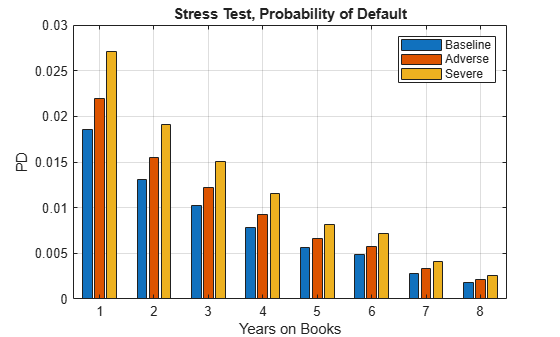### Lifetime PD and ECL

This section shows how to compute lifetime PDs using the Cox PH macro model and how to compute lifetime Expected Credit Losses (ECL).

For lifetime modeling, the PD model is the same, but it is used differently. You need the predicted PDs not just one period ahead, but for each year throughout the life of each particular loan. This means that you also need macro scenarios throughout the life of the loans. This section sets up alternative long-term macro scenarios, computes lifetime PDs under each scenario, and the corresponding 1-year PDs, marginal PDs, and survival probabilities. The lifetime and marginal PDs are visualized for each year, under each macro scenario. The ECL is then computed for each scenario and the weighted average lifetime ECL.

For concreteness, this section looks into an eight-year loan at the beginning of its third year and predicts the 1-year PD from years 3 through 8 of the life of this loan. This section also computes the survival probability over the remaining life of the loan. The relationship between the survival probability $\mathit{S}\left(\mathit{t}\right)$ and the 1-year PDs or hazard rates $\mathit{h}\left(\mathit{t}\right)$, sometimes also called the forward PDs, is:

`$\begin{array}{l}\mathit{S}\left(0\right)=1,\\ \mathit{S}\left(1\right)=\left(1-\mathit{h}\left(1\right)\right),\\ ...\\ \mathit{S}\left(\mathit{t}\right)=\mathit{S}\left(\mathit{t}-1\right)\left(1-\mathit{h}\left(\mathit{t}\right)\right)=\left(1-\mathit{h}\left(1\right)\right)\cdots \left(1-\mathit{h}\left(\mathit{t}\right)\right)\end{array}$`

For an example of this, see the Kaplan-Meier Method (Statistics and Machine Learning Toolbox).

The lifetime PD (LPD) is the cumulative PD over the life of the loan, given by the complement of the survival probability:

`$\mathrm{LPD}\left(\mathit{t}\right)=1-\mathit{S}\left(\mathit{t}\right)$`

Finally, another quantity of interest is the Marginal PD (MPD), which is the increase in the lifetime PD between two consecutive periods:

`$\mathrm{MPD}\left(\mathit{t}+1\right)=\mathrm{LPD}\left(\mathit{t}+1\right)-\mathrm{LPD}\left(\mathit{t}\right)$`

It follows that the Marginal PD is also the decrease in survival probability between consecutive periods, and also the hazard rate multiplied by the survival probability:

`$\mathrm{MPD}\left(\mathit{t}+1\right)=\mathit{S}\left(\mathit{t}\right)-\mathit{S}\left(\mathit{t}+1\right)=\mathit{h}\left(\mathit{t}+1\right)\mathit{S}\left(\mathit{t}\right)$`

Specify three macroeconomic scenarios, one baseline projection, and two simple shifts of 20% higher, or 20% lower values for the baseline growth, which are called 'faster growth' and 'slower growth', respectively. The scenarios in this example, and the corresponding probabilities, are simple scenarios for illustration purposes only. A more thorough set of scenarios can be constructed with more powerful models using Econometrics Toolbox™ or Statistics and Machine Learning Toolbox™; see for example Modeling the United States Economy (Econometrics Toolbox). Automated methods can usually simulate large numbers of scenarios. In practice, only a small number of scenarios is required. These scenarios and corresponding probabilities are selected combining quantitative tools and expert judgment.

```CurrentAge = 3; % currently starting third year of loan Maturity = 8; % loan ends at end of year 8 ScoreGroup = 'High Risk'; % High risk YOBLifetime = (CurrentAge:Maturity)'; NumYearsRemaining = length(YOBLifetime); tLifetime = (dataMacro.Year(end)+1:dataMacro.Year(end)+NumYearsRemaining)'; tLifetime0 = (dataMacro.Year(end):dataMacro.Year(end)+NumYearsRemaining)'; XPredictScore = ismember(ScoreGroupLabels(2:end)',ScoreGroup); XPredictScore = repmat(XPredictScore,NumYearsRemaining,1); GDPPredict = [2.3; 2.2; 2.1; 2.0; 1.9; 1.8]; GDPPredict = [0.8*GDPPredict GDPPredict 1.2*GDPPredict]; MarketPredict = [15; 13; 11; 9; 7; 5]; MarketPredict = [0.8*MarketPredict MarketPredict 1.2*MarketPredict]; ScenLabels = ["Slower growth" "Baseline" "Faster growth"]; NumMacroScen = size(GDPPredict,2); % Scenario probabilities, used for the computation of lifetime ECL PScenario = [0.2; 0.5; 0.3]; hPredict = zeros(size(GDPPredict)); for ii = 1:NumMacroScen XPredictLifetime = [XPredictScore GDPPredict(:,ii) MarketPredict(:,ii)]; hPredict(:,ii) = hCoxTD(YOBLifetime).*exp(XPredictLifetime*bCoxTD); end survivalLifetime = [ones(1,NumMacroScen); cumprod(1-hPredict)]; PDLifetime = 1-survivalLifetime; PDMarginal = diff(PDLifetime); figure; subplot(2,1,1) plot(tLifetime0,PDLifetime) xticks(tLifetime0) grid on xlabel('Year') ylabel('Lifetime PD') title('Lifetime PD By Scenario') legend(ScenLabels,'Location','best') subplot(2,1,2) bar(tLifetime,PDMarginal) grid on xlabel('Year') ylabel('Marginal PD') title('Marginal PD By Scenario') legend(ScenLabels)```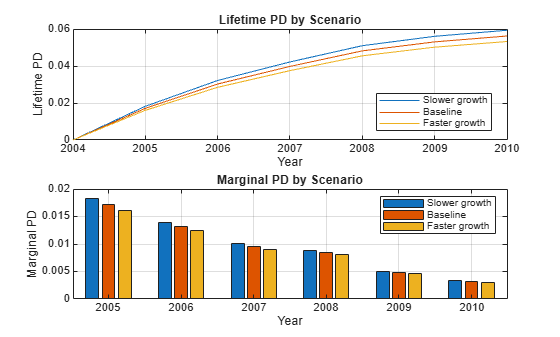These lifetime PDs by scenario are one of the inputs for the computation of lifetime expected credit losses (ECL), which also requires lifetime values for Loss Given Default (LGD) and Exposure at Default (EAD), for each scenario, and the scenario probabilities. For simplicity, in this example a constant LGD and EAD value is assumed, but these parameters could vary by scenario and by time period when LGD and EAD models are used.

The computation of lifetime ECL also requires the Effective Interest Rate (EIR) for discounting purposes. In this example, the discount factors are computed at the end of the time periods, but other discount times may be used, for example, the midpoint in between the time periods (that is, discount first-year amounts with a 6-month discount factor; discount second-year amounts with a 1.5-year discount factor, and so on).

With these inputs, the Expected Credit Loss at time t for scenario s is defined as:

`$\mathrm{ECL}\left(\mathit{t};\mathit{s}\right)=\mathrm{MPD}\left(\mathit{t};\mathit{s}\right)\text{\hspace{0.17em}}\mathrm{LGD}\left(\mathit{t};\mathit{s}\right)\text{\hspace{0.17em}}\mathrm{EAD}\left(\mathit{t};\mathit{s}\right)\text{\hspace{0.17em}}\mathrm{Disc}\left(\mathit{t}\right)$`

where t denotes a time period, s denotes a scenario, and $\mathrm{Disc}\left(\mathit{t}\right)=\frac{1}{{\left(1+\mathrm{EIR}\right)}^{\mathit{t}}}$.

For each scenario, a lifetime ECL is computed by adding ECLs across time, from the fist time period in the analysis, to the expected life of the product denoted by T, which in this example is five years (simple loan with five years remaining to maturity):

`$\mathrm{ECL}\left(\mathit{s}\right)=\sum _{\mathit{t}=1}^{\mathit{T}}\mathrm{ECL}\left(\mathit{t};\mathit{s}\right)$`

Finally, compute the weighed average of these expected credit losses, across all scenarios, to get a single lifetime ECL value, where $\mathit{P}\left(\mathit{s}\right)$ denotes the scenario probabilities:

`$\mathrm{ECL}=\sum _{\mathit{s}=1}^{\mathrm{NumScenarios}}\mathrm{ECL}\left(\mathit{s}\right)\mathit{P}\left(\mathit{s}\right)$`

```LGD = 0.55; % Loss Given Default EAD = 100; % Exposure at Default EIR = 0.045; % Effective Interest Rate DiscTimes = tLifetime-tLifetime0(1); DiscFactors = 1./(1+EIR).^DiscTimes; ECL_t_s = (PDMarginal*LGD*EAD).*DiscFactors; % ECL by year and scenario ECL_s = sum(ECL_t_s); % ECL total by scenario ECL = ECL_s*PScenario; % ECL weighted average over all scenarios % Arrange yearly ECL's for display in table format % Append ECL total per scenario, and scenario probabilities ECL_Disp = array2table([ECL_t_s; ECL_s; PScenario']); ECL_Disp.Properties.VariableNames = strcat("Scenario_",string(1:NumMacroScen)'); ECL_Disp.Properties.RowNames = [strcat("ECL_",string(tLifetime),"_s"); "ECL_total_s"; "Probability_s"]; disp(ECL_Disp)```
``` Scenario_1 Scenario_2 Scenario_3 __________ __________ __________ ECL_2005_s 0.94549 0.8921 0.84173 ECL_2006_s 0.71543 0.6789 0.64419 ECL_2007_s 0.53884 0.51412 0.49048 ECL_2008_s 0.40169 0.38527 0.36947 ECL_2009_s 0.20849 0.20098 0.19372 ECL_2010_s 0.12339 0.11952 0.11576 ECL_total_s 2.9333 2.7909 2.6554 Probability_s 0.2 0.5 0.3 ```
`fprintf('Lifetime ECL: %g\n',ECL)`
```Lifetime ECL: 2.77872 ```

When the LGD and EAD do not depend on the scenarios (even if they change with time), the weighted average of the lifetime PD curves can be taken to get a single, average lifetime PD curve.

```PDLifetimeWeightedAvg = PDLifetime*PScenario; ECLByWeightedPD = sum(diff(PDLifetimeWeightedAvg)*LGD*EAD.*DiscFactors); fprintf('Lifetime ECL, using weighted lifetime PD: %g, same result because of constant LGD and EAD.\n',... ECLByWeightedPD)```
```Lifetime ECL, using weighted lifetime PD: 2.77872, same result because of constant LGD and EAD. ```

However, when the LGD and EAD values change with the scenarios, you must first compute the ECL values at scenario level, and then find the weighted average of the ECL values.

### Conclusion

This example showed how to fit a Cox PH model for PDs, how to perform stress testing of the PDs, and how to compute lifetime PDs and ECL.

A similar example, Stress Testing of Consumer Credit Default Probabilities Using Panel Data, follows the same workflow but uses logistic regression, instead of Cox regression. The computation of lifetime PDs and ECL at the end of this example could be performed also with the logistic model, with some adaptations to the code.

You can modify the workflow presented in these two examples to use other model formulations. For example, you can fit the logistic model that treats the age as a categorical (discrete time) variable. In this case, the model PDs would more closely fit the observed PDs, but one would lose the extrapolation capabilities of the model. Moreover, instead of logistic regression, other generalized linear models (GLMs) supported by `fitglm` could also be used with minor changes to the code, for example, probit models or complementary log-log models. Because all of these models incorporate the age of the loan and macro information explicitly into the model, they can be used for stress testing and lifetime PD and ECL analysis.